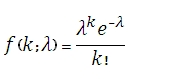# SQL Server POISSON.DIST function

Updated: 9 August 2010

Use POISSON to calculate the Poisson distribution. The formula for the probability density function (pdf) is:The formula for the cumulative distribution function (cdf) is:Where Q(a,x) is the regularized gamma function
Syntax
SELECT [wctStatistics].[wct].[POISSON] (
<@X, float,>
,<@Mean, float,>
,<@Cumulative, bit,>)
Arguments
@X
is the number of events. @X is an expression of type float or of a type that can be implicitly converted to float
@Mean
is the arithmetic mean of the distribution. @Mean is an expression of type float or of a type that can be implicitly converted to float
@Cumulative
is a logical value that determines if the probability density function (False, 0) or the cumulative distribution function (True, 1) is being calculated.
Return Types
float
Remarks
·         @X is truncated to zero decimal places.
·         If @X < 0, POISSON returns an error.
·         If @Mean < 0, POISSON returns an error.
Examples

select
wct.POISSON(2,5,'False')

This produces the following result

----------------------
0.0842243374885683

(1 row(s) affected)

select
wct.POISSON(2,5,'True')

This produces the following result

----------------------
0.124652019483081

(1 row(s) affected)

Select
wct.GAMMAQ(3, 5)

This produces the following result

----------------------
0.124652019483081

(1 row(s) affected)

### SupportCopyright 2008-2021 Westclintech LLC         Privacy Policy        Terms of Service